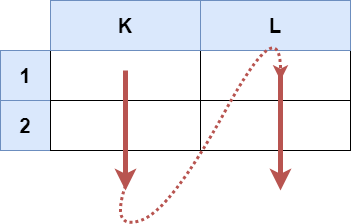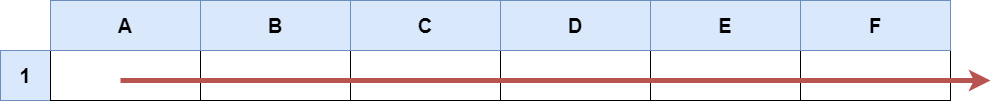##### Welcome to Subscribe On Youtube

Formatted question description: https://leetcode.ca/all/2194.html

# 2194. Cells in a Range on an Excel Sheet (Easy)

A cell (r, c) of an excel sheet is represented as a string "<col><row>" where:

• <col> denotes the column number c of the cell. It is represented by alphabetical letters.
• For example, the 1st column is denoted by 'A', the 2nd by 'B', the 3rd by 'C', and so on.
• <row> is the row number r of the cell. The rth row is represented by the integer r.

You are given a string s in the format "<col1><row1>:<col2><row2>", where <col1> represents the column c1, <row1> represents the row r1, <col2> represents the column c2, and <row2> represents the row r2, such that r1 <= r2 and c1 <= c2.

Return the list of cells (x, y) such that r1 <= x <= r2 and c1 <= y <= c2. The cells should be represented as strings in the format mentioned above and be sorted in non-decreasing order first by columns and then by rows.

Example 1:Input: s = "K1:L2"
Output: ["K1","K2","L1","L2"]
Explanation:
The above diagram shows the cells which should be present in the list.
The red arrows denote the order in which the cells should be presented.


Example 2:Input: s = "A1:F1"
Output: ["A1","B1","C1","D1","E1","F1"]
Explanation:
The above diagram shows the cells which should be present in the list.
The red arrow denotes the order in which the cells should be presented.


Constraints:

• s.length == 5
• 'A' <= s <= s <= 'Z'
• '1' <= s <= s <= '9'
• s consists of uppercase English letters, digits and ':'.

Similar Questions:

## Solution 1.

• // OJ: https://leetcode.com/problems/cells-in-a-range-on-an-excel-sheet/
// Time: O(1) since there are at most 26 * 9 cells.
// Space: O(1) extra space
class Solution {
public:
vector<string> cellsInRange(string s) {
vector<string> ans;
char a = s, b = s, x = s, y = s;
for (; a <= b; ++a) {
for (char i = x; i <= y; ++i) {
ans.push_back(string(1, a) + string(1, i));
}
}
return ans;
}
};

• class Solution:
def cellsInRange(self, s: str) -> List[str]:
return [
chr(i) + str(j)
for i in range(ord(s), ord(s[-2]) + 1)
for j in range(int(s), int(s[-1]) + 1)
]

############

# 2194. Cells in a Range on an Excel Sheet
# https://leetcode.com/problems/cells-in-a-range-on-an-excel-sheet

class Solution:
def cellsInRange(self, s: str) -> List[str]:
c1, r1, _, c2, r2 = s
res = []

for c in range(ord(c1), ord(c2) + 1):
for r in range(ord(r1), ord(r2) + 1):
res.append(chr(c) + chr(r))

return res


• class Solution {
public List<String> cellsInRange(String s) {
List<String> ans = new ArrayList<>();
for (char i = s.charAt(0); i <= s.charAt(3); ++i) {
for (char j = s.charAt(1); j <= s.charAt(4); ++j) {
}
}
return ans;
}
}

• func cellsInRange(s string) []string {
var ans []string
for i := s; i <= s; i++ {
for j := s; j <= s; j++ {
ans = append(ans, string(i)+string(j))
}
}
return ans
}


## Discuss

https://leetcode.com/problems/cells-in-a-range-on-an-excel-sheet/discuss/1823631